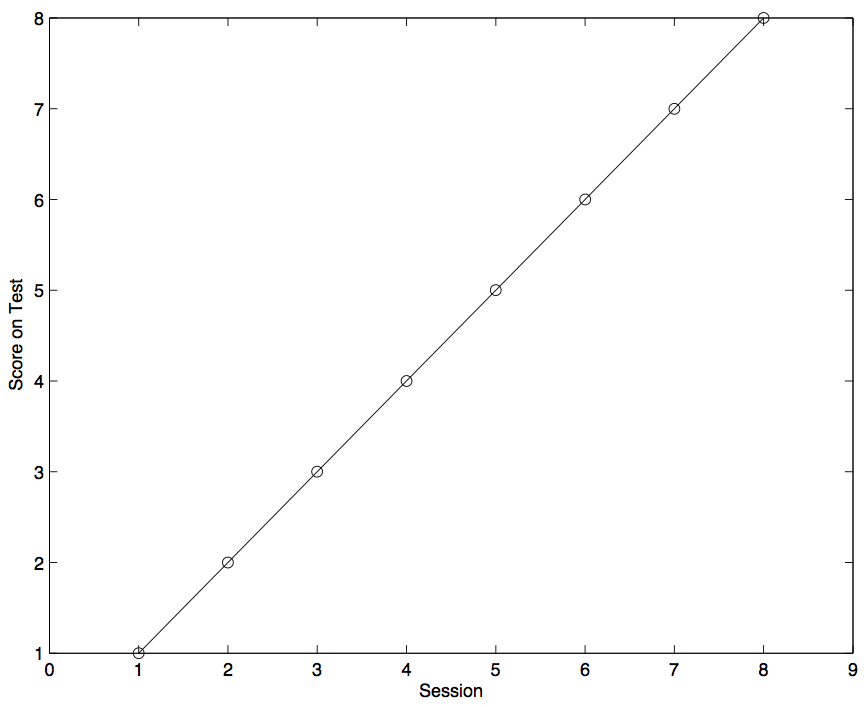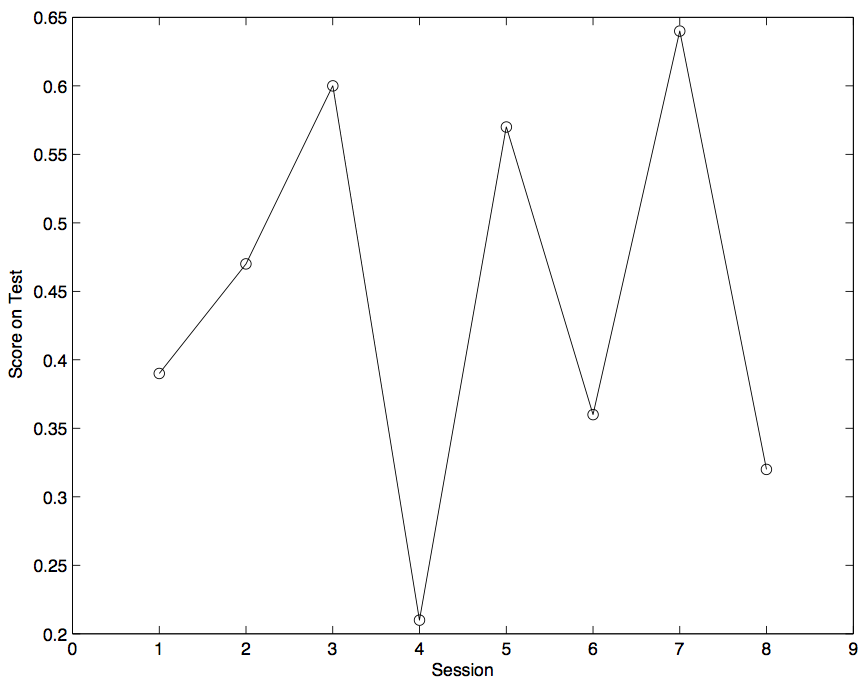### Code 1.8.1:

``````x = [1 2 3 4 5 6 7 8];
y = [0.39  0.47  0.60  0.21  0.57  0.36  0.64  0.32];
plot(x,x,'ko-')
xlim([0 9])
xlabel('Session')
ylabel('Score on Test')``````

### Output 1.8.1:### Code 1.8.2:

``````x = [1 2 3 4 5 6 7 8];
y = [0.39  0.47  0.60  0.21  0.57  0.36  0.64  0.32];
xlim([0 9])
xlabel('Session')
ylabel('Score on Test')``````

### Output 1.8.2### Code 1.11.1:

``````% Largest_So_Far_01

% Find the largest value in the one-row matrix V.
% Initialize largest_so_far to minus infinity.
% Then go through the matrix by first setting i to 1
% and then letting i increase to the value equal
% to the number of elements of V, given by length(V).
% If the i-th value of V is greater than largest_so_far,
% reassign largest_so_far as the i-th value of V.
% After going through the whole array, print out
% largest_so_far.

V = [7 33 39 26 8 18 15 4 0];
largest_so_far = -inf;
for i = 1:length(V)
if V(i) > largest_so_far
largest_so_far = V(i);
end
end
largest_so_far``````

### Output 1.11.1:

``````largest_so_far =
39``````

### Code 1.11.2:

``````% Largest_So_Far_02

% Find the largest value in the one-row matrix theDataArray.
% Initialize largest_so_far to minus infinity.
% Then go through the matrix, by first setting i to 1
% and then letting i increase to the value equal
% to the number of elements of theDataArray, given by
% length(theDataArray).
% If the i-th value of theDataArray is greater than
% largest_so_far,reassign largest_so_far with the i-th
% value of theDataArray.
% After having gone through the whole array, print out
% largest_so_far, which will be the largest value found.

theDataArray = [7 33 39 26 8 18 15 4 0];
largest_so_far = -inf;

for i = 1:length(theDataArray)
if theDataArray(i) > largest_so_far
%Got a new candidate!
largest_so_far = theDataArray(i);
end
end

% All done...so what's the maximum?
largest_of_them_all = largest_so_far``````

### Output 1.11.2:

``````largest_of_them_all =
39``````